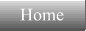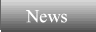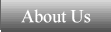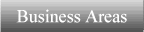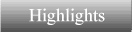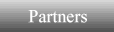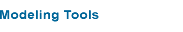AGPS also has a number of analysis tools that are not found in CAD systems. These permit a user to extract information from points, curves, and surfaces that can be used for evaluation or mathematical operations within the programming language.Routines to Interrogate GeometrySmoothing Algorithms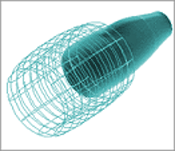Routines to Interrogate Geometry. AGPS contains a host of evaluation routines intended to provide high-level construct access to the building blocks of your geometry.Extract numerical value from an object Store the coordinates of point, curve, or surface objects Physical coordinates or parametric derivatives of an AGPS object Find the parameter value of a specified knot on a curve Find the outward normal unit vector of a surface Find the coefficients of a plane passing through three points Store the coordinates of an AGPS point-matrix Find the slope of a curve at a given parameter value Find the S- and T-parameter values at a given index of a surface Find the maximum chord height error between two parameter valuesExtract properties of an object Determine the index of an ancestor of an AGPS object Get the brand and brand-type system setting of an object Find the value of a particular descriptor of an AGPS object Find the number of ancestors of an AGPS object Return the name of an AGPS object Return Object Type Find a user qualified-name for a given AGPS object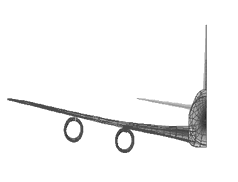Vector or Matrix Math operations Perform matrix addition, multiplication, copying, and, scaling. Clip matrix to an absolute value Dot product between two vectors Length of a vector Cross product between two vectors Normalize a vector Determine Eigenvalues Solve a system of linear equations Find the inverse and determinant of a matrixExtract information about the present mode of AGPS operation Return current level of numeric, character, and command keyword value tables. Flags the most recent error type that occurred. Find the dimensions of the current AGPS draw window Store the rotation angles, scale, translation, and perspective field of view angle Create interactive rubber-band window Create default or user specified color palletSmoothing AlgorithmsSmooth points, grids, and surface fitted grids by minimizing panel skewness Smooth an array of 2D or 3D points using 2D Thompson Grid Equations with orthogonality Smooth a lattice, list of arrays, or a point-matrix based upon 3D elliptic PDEs. Smooth an array based upon 2D elliptic PDEs. Smooth a specified sequence of points using a five-point smoother for a specified number of cycles Minimize the grid area of an existing array by modifying the interior pointsProduct Info Product Overview AGPS Capabilities Specifications Features Geometry System Modeling Tools Pre-Processing Programming Env. Data Translation Modules & Automation Advanced Visual. Tech. Briefs Solutions Case Studies Users Forums Support Info Request White Papers Release Notes